# Physics - Tangent Law - Superposition of Magnetic Fields

Tangent Law - Superposition of Magnetic Fields

Statement :

When a magnetic needle is acted upon by two magnetic fields at right angles to each other, the needle will be deflected through an angle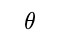such that the tangent of the angle of deflection is equal to the ratio of the magnetic fields.

Mathematically,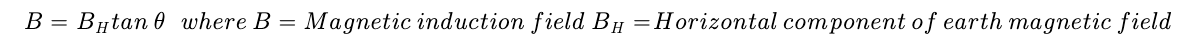Explanation

Let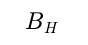and B be the earth's and applied magnetic field of induction which are perpendicular to each other.If a freely suspended magnetic needle is placed in these field, the needle comes to rest in the resultant field direction shown in figure.

If the needle makes an anglewith earth's magnetic induction then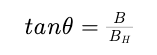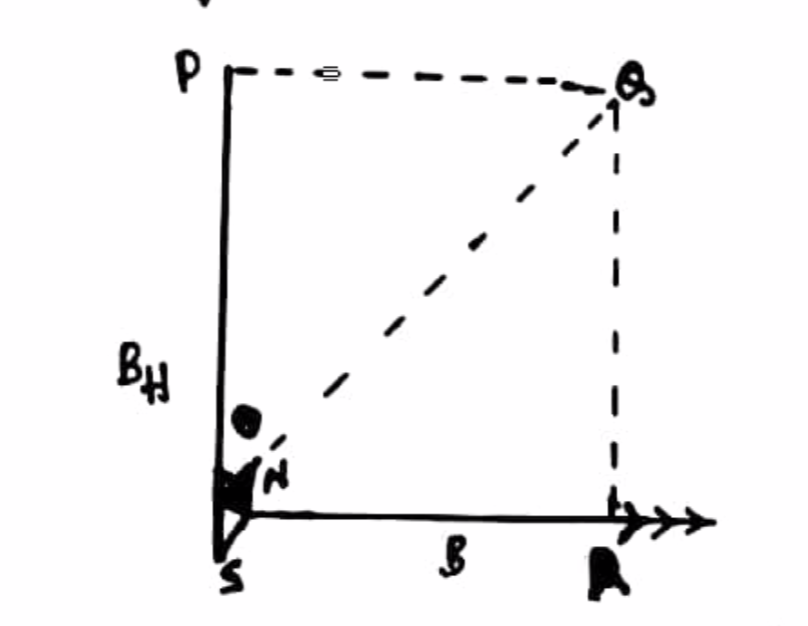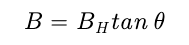The above equation is called Tangent Law.

Post By : Ashish Maurya 17 May, 2020 485 views Physics